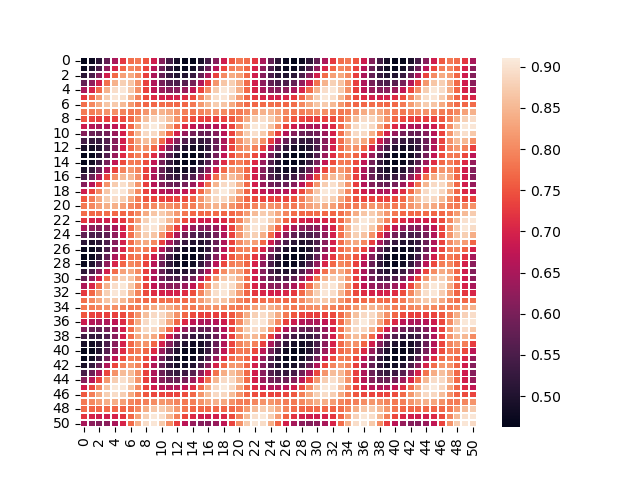# How to perform change of basis from a non-orthogonal 3D array to an orthogonal 3D array ?

I am trying to understand how to code the resampling of the voxels from a non-orthogonal basis as shown in the image below (taken from SimpleITK’s homepage) to an orthogonal grid.

My input is say a 3D grayscale image/3D NumPy array (in a non-orthogonal grid) of dimensions MxMxM and I want to resample it onto an orthogonal grid of any dimensions (3D NumPy array). Can you tell me if it can be achieved by SimpleITK’s functions as well.

An example of a 2D slice (heatmap) of my 3D inputs is shown below. Basically those elliptical objects you see, will become circular post change of basis.Hello @pranoy-ray,

Yes, this is readily done using SimpleITK.

For specific code, please see the `make_isotropic` function in this jupyter notebook.

@zivy Thank You for your reply, but how do I cast a 3D NumPy array to an Sitk object, which can be passed it into the make_isotropic function?

Hello @pranoy-ray,

To get a SimpleITK image from a numpy array:

``````image = sitk.GetImageFromArray(numpy_array)
``````

For full details see this jupyter notebook.

This will assume orthogonal axes. You need to provide the actual vectors via `SetDirection()`.

Hi @dzenanz @zivy I did what you guys said, but it doesn’t seem to do the basis transformation.

Assuming “data_3d” is the 3D NumPy array containing my 3D image (Primitive Cell FCC Lattice-Voxelized), my code is as follows:

``````image = sitk.GetImageFromArray(data_3d)
image.SetDirection(tuple((0.5, 0.5, 0.0, 0.0, 0.5, 0.5, 0.5, 0.0, 0.5)))
un=make_isotropic(image)
sitk.Show(un,"Final Data")
``````

Trying to make the transformation from non-orthogonal basis (60,60,60) to orthogonal basis (90,90,90)

Please can you tell me where I’m going wrong?